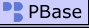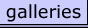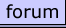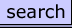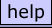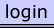Jean-Marie Choisel | all galleries >> FRACTALES / FRACTALS >> FRACTINT - TYPE : COMPLEX MARKS JULIA > FRACTINTTYPE COMPLEX MARKSJULIACXMJ010
 previous | next
 2014/08/23 FRACTINT / Jean-Marie CHOISEL

### FRACTINTTYPE COMPLEX MARKSJULIACXMJ010

Fractal type : complex marksjulia.
This is a generalization of marksjulia fractals.

Basic equations provided by FRACTINT for this type of fractals :

z(n) = pixel;
z(n+1) = (c^(p-1)) * z(n-1)^2 + c

Four parameters : real and imaginary parts of c and p.
The default bailout value is 4.

For the factal here, the following parameters are used :
real(c) = 0.3, imag(c) = 0.5
real(p) = 1, imag(p) = 0
Bailout test = mod (bailout test can take one of the 7 following values : mod, real, imag, or, and, manh manr)
Bailout value = 8 (this means that if z(n) = z(x + iy) then |z| = sqrt(x^2 + y^2) >= bailout value (here 8).
Maximum number of iterations = 150
Inside color = 1 (7 values available : , iter, real, imag, mult, summ, atan)
Outside color = iter
Potential Max Color = 255
Slope = 0
Bailout = 0
Disyance Estimator = 0
Width factor = 0